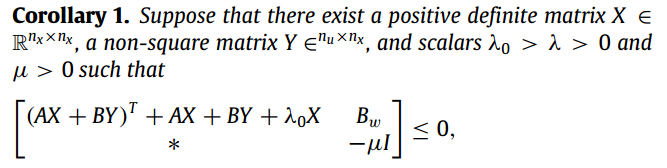# How to solve this LMII want to obtain X,Y and mu in the picture. My code is attached bellow.

A=[-1,2;-3,4];B=[0.5;-2];Bw=[0;1];
cvx_begin sdp
variable X(2,2) symmetric;variable Y(1,2);variable lambda0;variable mu1;
subject to
[(A*X+B*Y)'+A*X+B*Y+lambda0*X,Bw;Bw',-mu1*eye(1)]<=0;
X>=0;lambda0>=0;mu1>=0;
cvx_end


Disciplined convex programming error:
Invalid quadratic form(s): not a square.
It shows the error above and I find out lambda0*X goes wrong. But How can I solve this problem?

That error is because this is NOT an LMI due to the product term \lambda_0 X. It is a BMI (Bilinear Matrix Inequality), which is non-convex and will not be accepted by CVX. Why isn't CVX accepting my model? READ THIS FIRST!.

That said, I think you can solve this feasibility problem using the methods in section 4.2.5 “Quasiconvex optimization” in “Convex Optimization” by Boyd and Vandenberghe http://web.stanford.edu/~boyd/cvxbook/ . I leave you to work out the details.

Note that as a practical matter to deal with strict inequalities in the Corollary statement, and avoid the zero (singular for X) solution, you will need to use something like

X >= small_number_1*eye(n_x)
lambda0 >= small_number_2
mu1 > =small_number_3


where small_number_1, small_number_2, small_number3 are small positive numbers, which are very small, but larger than solver feasibility tolerance. So perhaps 1e-6 or 1e-5.

1 Like